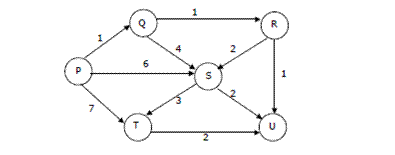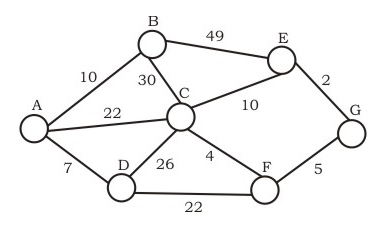# Exercise: Questions on Greedy Algorithms

Questions on Greedy Algorithms : Question 1 :
Which of the following standard algorithms is not a Greedy algorithm?

 Dijkstra's shortest path algorithm Prim's algorithm Kruskal algorithm Huffman Coding Bellmen Ford Shortest path algorithm  Correct
Show Answer
Questions on Greedy Algorithms : Question 2 :
Suppose we run Dijkstraâs single source shortest-path algorithm on the following edge weighted directed graph with vertex P as the source. In what order do the nodes get included into the set of vertices for which the shortest path distances are finalized? (GATE CS 2004)P, Q, R, S, T, U P, Q, R, U, S, T  Correct P, Q, R, U, T, S P, Q, T, R, U, S
Show Answer
Questions on Greedy Algorithms : Question 3 :
A networking company uses a compression technique to encode the message before transmitting over the network. Suppose the message contains the following characters with their frequency:
```character   Frequency
a	        5
b           9
c           12
d           13
e           16
f           45
```
Note : Each character in input message takes 1 byte. If the compression technique used is Huffman Coding, how many bits will be saved in the message?

 224 800 576  Correct 324
Show Answer
Questions on Greedy Algorithms : Question 4 :
What is the time complexity of Huffman Coding?

 O(N) O(NlogN)  Correct O(N(logN)^2) O(N^2)
Show Answer
Questions on Greedy Algorithms : Question 5 :
In question #2, which of the following represents the word "dead"?

 1011111100101 0100000011010 Both A and B  Correct None of these
Show Answer
Questions on Greedy Algorithms : Question 6 :
Which of the following is true about Kruskal and Prim MST algorithms? Assume that Prim is implemented for adjacency list representation using Binary Heap and Kruskal is implemented using union by rank.

 Worst case time complexity of both algorithms is same.  Correct Worst case time complexity of Kruskal is better than Prim Worst case time complexity of Prim is better than Kruskal
Show Answer
Questions on Greedy Algorithms : Question 7 :
Which of the following is true about Huffman Coding.

 Huffman coding may become lossy in some cases Huffman Codes may not be optimal lossless codes in some cases In Huffman coding, no code is prefix of any other code.  Correct All of the above
Show Answer
Questions on Greedy Algorithms : Question 8 :
Suppose the letters a, b, c, d, e, f have probabilities 1/2, 1/4, 1/8, 1/16, 1/32, 1/32 respectively. Which of the following is the Huffman code for the letter a, b, c, d, e, f?

 0, 10, 110, 1110, 11110, 11111  Correct 11, 10, 011, 010, 001, 000 11, 10, 01, 001, 0001, 0000 110, 100, 010, 000, 001, 111
Show Answer
Questions on Greedy Algorithms : Question 9 :
Suppose the letters a, b, c, d, e, f have probabilities 1/2, 1/4, 1/8, 1/16, 1/32, 1/32 respectively. What is the average length of Huffman codes?

 3 2.1875 2.25 1.9375  Correct
Show Answer
Questions on Greedy Algorithms : Question 10 :
Consider the undirected graph below:Using Prim's algorithm to construct a minimum spanning tree starting with node A, which one of the following sequences of edges represents a possible order in which the edges would be added to construct the minimum spanning tree?

 (E, G), (C, F), (F, G), (A, D), (A, B), (A, C) (A, D), (A, B), (A, C), (C, F), (G, E), (F, G) (A, B), (A, D), (D, F), (F, G), (G, E), (F, C) (A, D), (A, B), (D, F), (F, C), (F, G), (G, E)  Correct
Show Answer